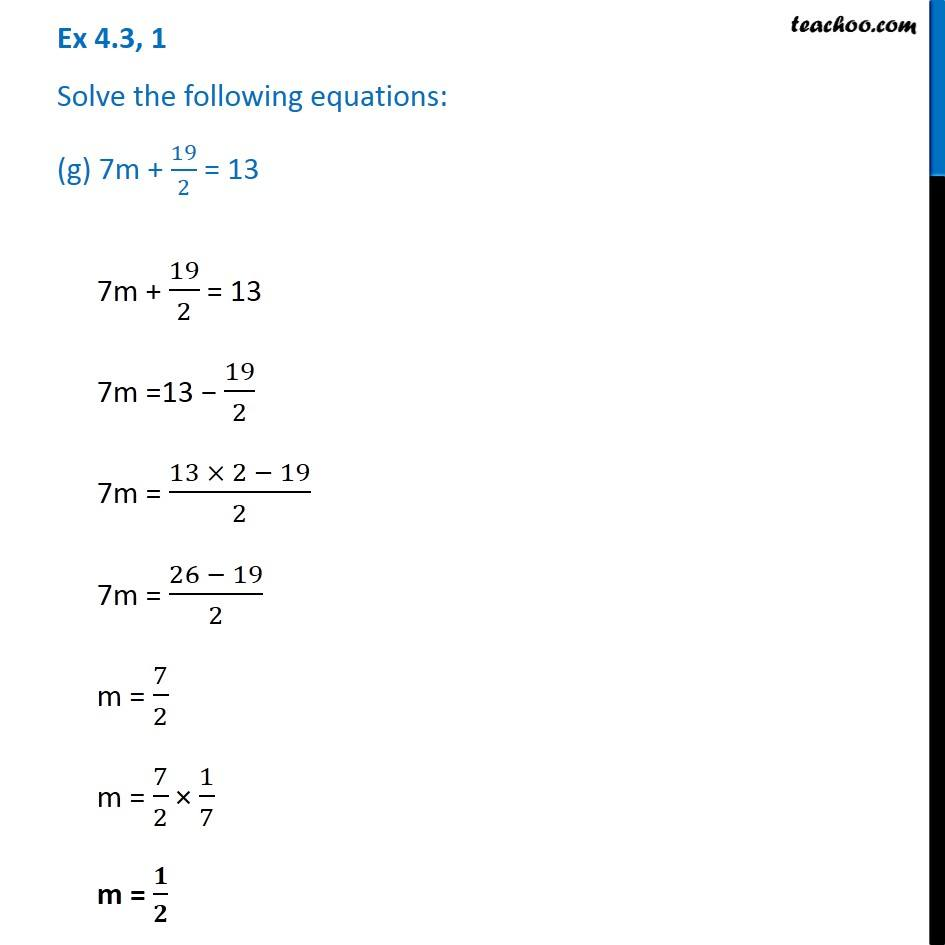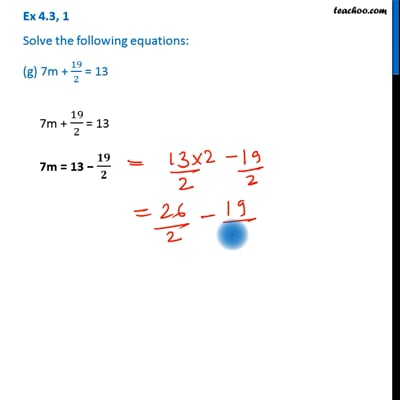Ex 4.3

Chapter 4 Class 7 Simple Equations
Serial order wiseThis video is only available for Teachoo black users

Get live Maths 1-on-1 Classs - Class 6 to 12

### Transcript

Ex 4.3, 1 Solve the following equations: (g) 7m + 19/2 = 137m + 19/2 = 13 7m =13 − 19/2 7m = (13 × 2 − 19)/2 7m = (26 − 19)/2 m = 7/2 m = 7/2 × 1/7 m = 𝟏/𝟐